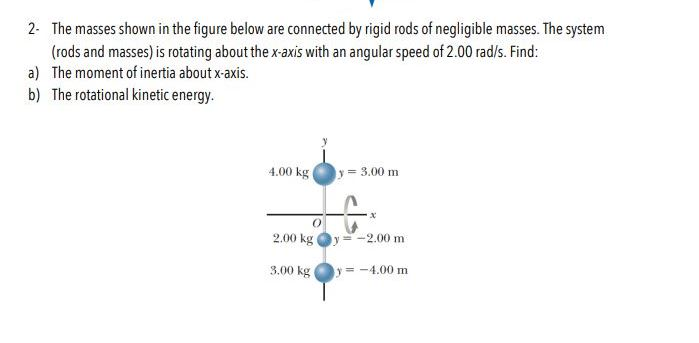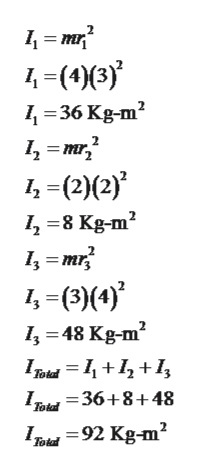# 2 The masses shown in the figure below are connected by rigid rods of negligible masses. The system(rods and masses) is rotating about the x-axis with an angular speed of 2.00 rad/s. Find:a) The moment of inertia about x-axis.b) The rotational kinetic energy4.00 kg= 3.00 m-2,00 m2.00 kg=-4.00 m3.00 kg

Question
3 viewshelp_outlineImage Transcriptionclose2 The masses shown in the figure below are connected by rigid rods of negligible masses. The system (rods and masses) is rotating about the x-axis with an angular speed of 2.00 rad/s. Find: a) The moment of inertia about x-axis. b) The rotational kinetic energy 4.00 kg = 3.00 m -2,00 m 2.00 kg =-4.00 m 3.00 kg fullscreen
check_circle

star
star
star
star
star
1 Rating
Step 1

Given,

Step 2

Part (a):

Moment of Inertia about x-axis can be calculated as,

Step 3

By substituting the given values moment of...help_outlineImage Transcriptionclosem 4 36 Kg-m2 /-(2)(2) 8 Kg-m2 mr -(3)4) 48 Kg-m2 I 36+8+48 Totd Totd fullscreen

### Want to see the full answer?

See Solution

#### Want to see this answer and more?

Solutions are written by subject experts who are available 24/7. Questions are typically answered within 1 hour.*

See Solution
*Response times may vary by subject and question.
Tagged in

### Angular Motion